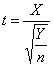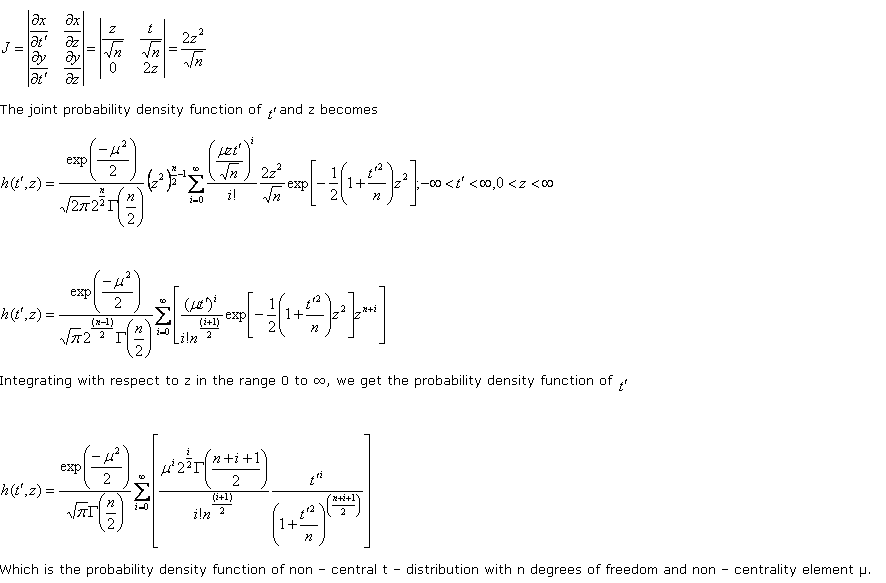# Statistics Assignment Help With Non Central T DistributionThe non – central t – distribution is the distribution of the ratio of a normal variate with possibly non – zero mean and variate unity, to the square root of an independent χ2 – variate divided by its degrees of freedom. If X ≈ N(μ, 1) and Y is an independent χ2 – variate with n degrees of freedom thenIs said to have a non – central t – distribution with n degrees of freedom and non – centrality parameter μ. Non – central t – distribution is required for the power functions of certain tests concerning normal population.

Probability density function of ‘t’. since X ≈ N(μ, 1)

###Which is the probability density function of non – central t – distribution with n degrees of freedom and non – centrality element µ.